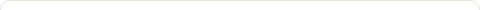# Java Program to convert Decimal to Binary

Posted on: March 15, 2016

Objectives :

• Decimal to Binary Conversion
• Converting Decimal to Binary
• Converting Decimal Number to Binary format
• Write a program to convert decimal to binary
• Write a program to convert decimal number to binary format
• Write a program to convert positive decimal number to binary format
• Write a program to convert decimal to binary using Scanner class
• Write a program to convert decimal to binary, take input using Scanner class

Java Program to convert Decimal to Binary :

```public class DecimalToBinary {

public void toBinary(int number){
int binary[] = new int;
int n = 0;
// Convert Decimal to Binary
while(number > 0){
binary[n++] = number%2;
number = number/2;
}
// Print Binary number
for(int i = n-1;i >= 0;i--){
System.out.print(binary[i]);
}
}

public static void main(String[] args){
DecimalToBinary obj = new DecimalToBinary();
obj.toBinary(14);
}
}
```

Output :

1110

Java Program to convert Decimal to Binary using Scanner class :

```
import java.util.Scanner;

public class DecimalToBinary {

public void toBinary(int number){
int binary[] = new int;
int n = 0;

// Convert Decimal to Binary
while(number > 0){
binary[n++] = number%2;
number = number/2;
}

// Print Binary Number
for(int i = n-1;i >= 0;i--){
System.out.print(binary[i]);
}
}

public static void main(String[] args) {
Scanner scanner = new Scanner(System.in);
System.out.print("Enter a number: ");
int num= scanner.nextInt();
DecimalToBinary obj = new DecimalToBinary();
obj.toBinary(num);

}
}

```

Output :

Enter a number: 14

1110

### 1 Response to "Java Program to convert Decimal to Binary"You explained very well by using above simple examples of how to convert decimal number to binary number in java.

Like